# 3rd Grade Math Worksheets: Division

## Free division worksheets

Our 3rd grade division worksheets include i) simple division worksheets to help kids with their division facts and mental division skills and ii) an introduction to long division including simple division with remainder questions.  Practice dividing by tens and hundreds is also emphasized.

## Meaning of division

Division sentences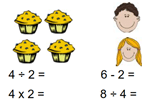Dividing into equal groups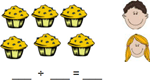## Division

Division by 2 or 3 9 ÷ 3 =
Division by 4 or 5 10 ÷ 5 =
Division by 6 or 7 12 ÷ 6 =
Division by 8 or 9 16 ÷ 8 =

Division facts practice (tables 1-10) 54 ÷ 9 =
Division facts practice (tables 1-12) 33 ÷ 11 =

Missing dividend or divisor (basic facts, 1-10) ___ ÷ 5 = 3
Missing dividend or divisor (basic facts, 1-12) ___ ÷ 12 = 5
Multiplication and division fact families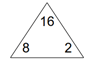Divide by 10 340 ÷ 10 =
Divide by 100 4,400 ÷ 100 =
Divide by whole tens 5,400 ÷ 90 =
Divide by whole hundreds 5,600 ÷ 800 =

Divide 3 or 4-digit numbers by 1-digit numbers 2,800 ÷ 4 =
Division with remainder within 1-100, based on basic facts 51 ÷ 8 =

## Long division

Division facts practice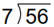Division with remainder, within 0-100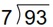## Division word problems